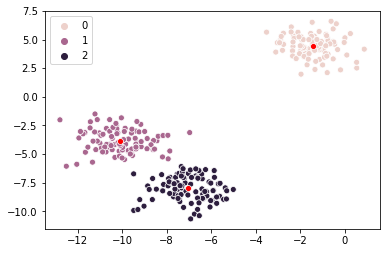# Data Science Bootcamp

During the Summer of 2019 I taught a bootcamp of 432 hours on Data Science at NEOLAND with the following contents:

1. Matematical foundations. Basic notions of Probability and Statistics. Elementary Linear Algebra. Multivariable Calculus.
2. Practical programming. Introduction to Python. Numpy. Pandas. Input/Output libraries. Introduction to R.
3. Data visualization. Matplotlib. Seaborn.
4. Data mining. Twitter API. Scrapping. Machine Learning. Scikit-Learn. Linear Regression. Logistic Regression. Naive Bayes. K Nearest Neighbours. Singular Value Decomposition and Principal Component Analysis. Support Vector Machines. K Means. Trees. Ensemble Learning. Natural Language Processing. Sentiment analysis. Deep Learning. Neural nets. TensorFlow. Keras.
5. Database systems. MySQL. SQLite3. MongoDB.
6. Final project.

Here are some useful references:

Here is some material that I developed for the course (in Spanish):